GRE Subject Test: Biochemistry, Cell, and Molecular Biology : Help with Hardy-Weinberg

Example Questions

← Previous 1

Example Question #1 : Inheritance

A population of insects exists in which black coloration is dominant to white. If there are 64 black insects and 36 white insects in the population, what is the recessive allele frequency?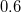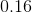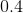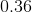Explanation:

We can use the white insect population to figure out our recessive allele frequency if we use the Hardy-Weinberg equations: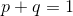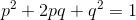From this equation we know that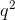is actually the frequency of our homozygous recessive genotype. We can determine the value of this genotypic frequency based on the information in the question. Any white insects must be homozygous recessive.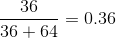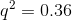Solve for the recessive allele frequency.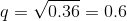Example Question #2 : Inheritance

A population of lizards is shown to have 36 members that are homozygous dominant, 48 members that are heterozygous, and 16 members that are homozygous recessive for a particular trait. The population displays Hardy-Weinberg equilibrium. What are the allele frequencies present in this population?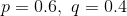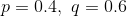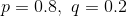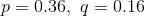Explanation:

To solve this question, we must use the Hardy-Weinberg equations:There are two ways to solve this problem. The easier way is to use the second Hardy-Weinberg equation. We are told that the population is in Hardy-Weinberg equilibrium, so the observed genotype frequencies equal the expected genotype frequencies. Each term in the second Hardy-Weinberg equation can be used in coordination with the given phenotypic frequencies.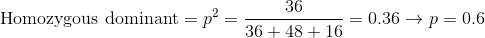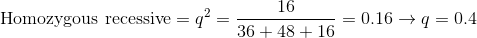The dominant allele frequency is 0.6 and the recessive allele frequency is 0.4.

The second method involves using our population and the total number of alleles. Since our population totals to 100, we have a total of 200 alleles (two alleles per member in the population. Next, to get the frequencies we simply have to divide the total number of a single allele by the total number of alleles in the population. For the dominant allele frequency it would look like this: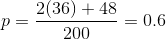We can then get the recessive allele frequency from the first Hardy-Weinberg equation: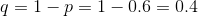Both methods result in the same answer.

Example Question #3 : Help With Hardy Weinberg

A population of beetles exists in which black coloration is dominant to white. The allele frequencies of the population were originally o.4 for the dominant allele and 0.6 for the recessive allele. A predator was introduced that selectively ate the white beetles. The new population consists of 36 homozygous dominant black beetles, 48 heterozygous beetles, and 16 white beetles. What are the new allele frequencies?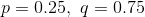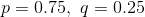Explanation:

The original allele frequencies are actually superfluous information that we will not need to use in our calculation. We are given the populations of each genotype, so we can use the Hardy-Weinberg equations to solve for the allele frequencies.In the second equation,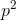gives the frequency of the homozygous dominant phenotype andgives the frequency of the homozygous recessive phenotype. Using the population ratios from the question we can solve for these values to find the allele frequencies.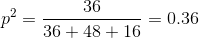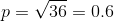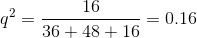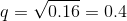The problem could also be solved by summing the total number of alleles, and dividing the total of each individual allele by this number. Keep in mind that each individual carries two alleles.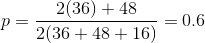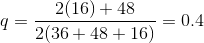Example Question #4 : Help With Hardy Weinberg

Which of the following choices are likely to change the allele frequencies of the indicated populations?

I. A geographic barrier isolating a small subset of a larger population

II. The introduction of a predator that only preys upon the homozygous dominant members of the population

III. A population that displays completely random mating

II only

III only

I, II, and III

I and II

I and II

Explanation:

Allele frequencies are the measure of an allele in relation to the total number of alleles in the given population. Introducing a predator that only preys upon homozygous dominant members will cause the number of dominant alleles to drop significantly and will, therefore, change allele frequencies. This would be an example of the bottleneck effect. Isolating a small subset of a population is going to change allele frequencies because that small subset is not likely to accurately represent the original population. This is an example of the founder effect.

Random mating is actually a factor that helps maintain allele frequencies, and is a requirement for Hardy-Weinberg equilibrium.

Example Question #1 : Help With Hardy Weinberg

Which of the following is not a condition for Hardy-Weinberg equilibrium?

Negligible mutation frequencies

Large population size

Completely random mating

Natural selection is operating on the population

Natural selection is operating on the population

Explanation:

Of the choices, the only one that is not a Hardy-Weinberg assumption is that natural selection is occurring on the population. In fact, the exact opposite is a Hardy-Weinberg assumption. If natural selection is occurring on a population, over a large period of time, it is likely to have an effect on allele frequencies within the population.

All other answers are requirements in order for Hardy-Weinberg equilibrium to be in effect: large population size, random mating, and negligible mutation frequencies.

Example Question #2 : Help With Hardy Weinberg

Which of the following conditions are not necessary for a population to be in Hardy-Weinberg equilibrium?

No migration between populations occurs

Mating must happen at random

There are no mutations

Natural selection affects the alleles under consideration

Population size must be large

Natural selection affects the alleles under consideration

Explanation:

The Hardy-Weinberg equilibrium states that the frequency of alleles at a locus remains constant from generation to generation. In order for this to be the case, natural selection cannot affect the alleles under consideration. All other answer choices describe conditions that do need to be met for Hardy-Weinberg equilibrium to be displayed. Note that the conditions for Hardy-Weinberg equilibrium are not met in nature.

Example Question #1 : Help With Hardy Weinberg

An isolated population consists of 10 males and 10 females. Two individuals are carriers of the recessive blue eye allele. Assuming all Hardy-Weinberg conditions are met. What is the frequency of the blue eye phenotype in the population?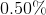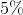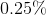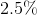Explanation:

Use the two Hardy-Weinberg equations:Above,is the frequency of the dominant allele, and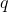is the frequency of the recessive allele in the isolated population.

Since there are 20 people in total on the island, that means that there are 40 alleles for eye color. 2 of the 40 are for the blue allele: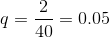We are looking for the blue eye phenotype, which can only result from two recessive alleles.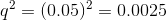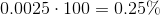Example Question #8 : Help With Hardy Weinberg

Within his rat population, a scientist is trying to generate twice as many recessive homozygotes as heterozygotes. What allelic frequency would accomplish this?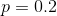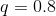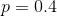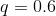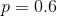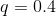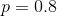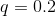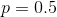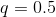Explanation:

Use the Hardy-Weinberg equations: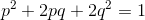The equation he will need to set up is the following: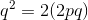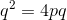Solve forand substitute the first equation into the equation above.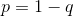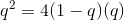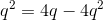Simplify.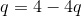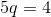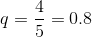Lastly, find.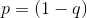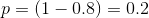Example Question #9 : Help With Hardy Weinberg

Assuming Hardy-Weinberg equilibrium conditions, what are the heterozygote (Bb) and homozygote recessive (bb) genotypes for a gene if the homozygote dominant (BB) genotype is 0.45?

Bb = 0.11

bb = 0.44

Bb = 0.4

bb = 0.15

Cannot be determined

Bb = 0.15

bb = 0.4

Bb = 0.44

bb = 0.11

Bb = 0.44

bb = 0.11

Explanation:

The correct answer is Bb = 0.44 and bb = 0.11.

Since we know BB = 0.45 and the equations for allele frequencies when Hardy-Weinberg equilibrium conditions are met: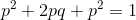andWe solve for B first: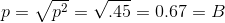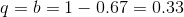Now we can solve for the homozygote recessive.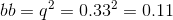Lastly, solve for the heterozygote.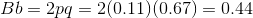Example Question #10 : Help With Hardy Weinberg

Which of the following is not an assumption of the Hardy-Weinberg equilibrium?

No natural selection within a population

Non-random mating within a population

No mutations within a population

No genetic drift within a population

No gene flow between populations